/

### The generalised inverse Gaussian distribution

Distribution name

Generalised inverse Gaussian distribution (GIG)

Common notation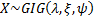Parameters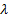= parameter (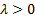)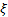= parameter (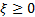)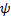= parameter (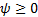)

Domain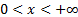Probability density function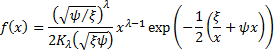where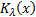is the modified Bessel function of the third kind with index.

Mean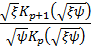Variance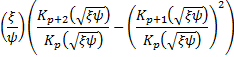Characteristic function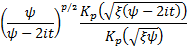When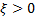and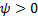the non-central moments are: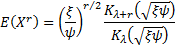Some commentators use GIG to refer to the generalised integer gamma distribution (which is not the same as the generalised inverse Gaussian distribution).

Nematrian web functions

This distribution is not currently supported within the Nematrian web function library. For details of other supported probability distributions see here.